## Example Questions

### Example Question #1 : Simplifying

Solve for x when 6x – 4 = 2x + 5

9/4

3

0

1/4

9/4

Explanation:

Solve by simplifying:

6x – 4 = 2x + 5

6x = 2x + 9

4x = 9

x = 9/4

### Example Question #2 : Simplifying

What is the value of the following equation if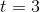and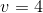?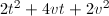Explanation:

Substitute the numbers 3 and –4 for t and v, respectively.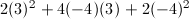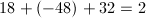### Example Question #3 : Simplifying

Simplify the following binomial: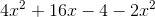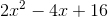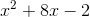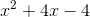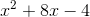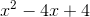Explanation:

The equation that is presented is:To get the correct answer, you first need to combine all of the like terms. So, you can subtract the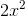from the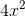, leaving you with: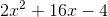From there, you can reduce the numbers by their greatest common denominator, in this case,:### Example Question #4 : Simplifying

Simplify the following binomial expression: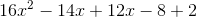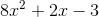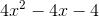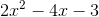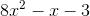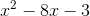Explanation:

First, combine all of the like terms that you are able: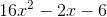Then, reduce by the greatest common denominator (in this case,):### Example Question #5 : Simplifying

Simplify the following binomial: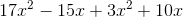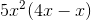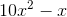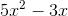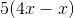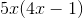Explanation:

The equation presented in the problem is:First you have to combine the like terms, i.e. combining all instances ofand: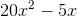Then, you can factor out the common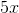to get your answer### All ACT Math Resources